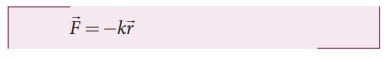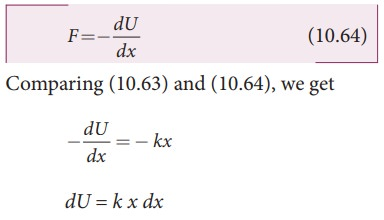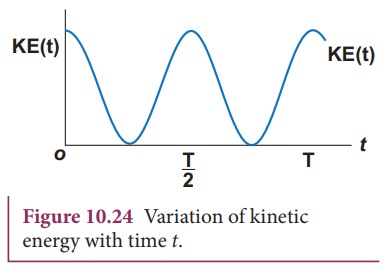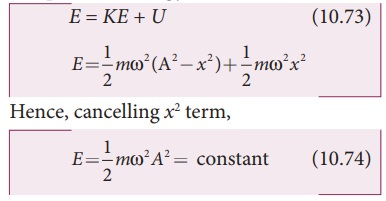Home | | Physics 11th std | Energy in Simple Harmonic Motion

# Energy in Simple Harmonic Motion

a. Expression for Potential Energy b. Expression for Kinetic Energy c. Expression for Total Energy

ENERGY IN SIMPLE HARMONIC MOTION

## a. Expression for Potential Energy

For the simple harmonic motion, the force and the displacement are related by Hooke’s lawSince force is a vector quantity, in three dimensions it has three components. Further, the force in the above equation is a conservative force field; such a force can be derived from a scalar function which has only one component. In one dimensional caseAs we have discussed in unit 4 of volume I, the work done by the conservative force field is independent of path. The potential energy U can be calculated from the following expression.

Comparing (10.63) and (10.64), we getThis work done by the force F during a small displacement dx stores as potential energyFrom equation (10.22), we can substitute the value of force constant k = m ω2 in equation (10.65),where ω is the natural frequency of the oscillating system. For the particle executing simple harmonic motion from equation (10.6), we get

x = A sin ωt## b. Expression for Kinetic Energy

Kinetic energySince the particle is executing simple harmonic motion, from equation (10.6)

x = A sin ωtTherefore, velocity isThis variation with time is shown below.## c. Expression for Total Energy

Total energy is the sum of kinetic energy and potential energyAlternatively, from equation (10.67) and equation (10.72), we get the total energy as

E =1/2 mω 2 A 2 sin 2 ωt + 1/2 mω 2 A2 cos2 ωt

= 1/2 mω 2 A2 (sin2 ω t +cos2 ωt)

From trigonometry identity,

(sin2ωt + cos2ωt) = 1

E = 1/2 mω2 A2 = constant

which gives the law of conservation of total energy. This is depicted in Figure 10.26Thus the amplitude of simple harmonic oscillator, can be expressed in terms of total energy.### EXAMPLE 10.15

Write down the kinetic energy and total energy expressions in terms of linear momentum, For one-dimensional case.

### Solution

Kinetic energy is KE= 1/2 mvx2

Multiply numerator and denominator by m

KE= [1/2m] m2 vx2 = [1/2m] (mvx )2 = [1/2m] px2

where, px is the linear momentum of the particle executing simple harmonic motion.

Total energy can be written as sum of kinetic energy and potential energy, therefore, from equation (10.73) and also from equation (10.75), we get

E= KE +U( x) = [1/2m] px2 + 1/2 mω2 x2 = constant### EXAMPLE 10.16

Compute the position of an oscillating particle when its kinetic energy and potential energy are equal.

### Solution

Since the kinetic energy and potential energy of the oscillating particle are equal,

1/2 mω 2 (A2 x 2 ) = 1/2 mω2 x2

A2 x2 = x22x2 = A2

x = ±A/√2

Study Material, Lecturing Notes, Assignment, Reference, Wiki description explanation, brief detail
11th Physics : UNIT 10 : Oscillations : Energy in Simple Harmonic Motion |